Friday , March 22 2019
Home / Profit and Loss: Problems Based On Successive/Consumption/Expenditure Concepts# Profit and Loss: Problems Based On Successive/Consumption/Expenditure Concepts

### Profit and Loss Problems: Different Types of questions that feature in the topic

In this article, we simply cover the different types of Profit and Loss problems that are based on the concepts of Profit and Loss. In this article, we do not cover any concepts as such and simply focus on the different problem types that feature in various examinations. In this article, you simply practice the different Profit and Loss problem types. For concept articles, refer to the three previous articles for this topic.

#### Type-1: Consumption – expenditure based

If the price of an item increases by r% ,then the percentage reduction in consumption so that expenditure remains the same ,is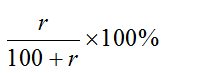Question 1: If price of sugar increases by 20% ,then the percentage reduction in consumption so that expenditure remains the same will be ?

Solution: To find the percentage reduction in consumption, apply formula(20/120)x100=50/3%

#### Type-2:  If the price of a commodity decreases by r% then percentage increase in consumption , so as not to decrease expenditure on this item is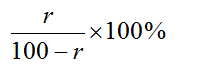Question 2:  If the price of sugar falls down by 10% ,by how much percent must a householder increase its consumption , so as not  to decrease expenditure in this item ?

Solution: To find the percentage increase in consumption, apply formula:-{10/(100-10)} x 100 = 100/9%

#### Type-3: If a reduction of x% in price enables a person to buy y kg more for Rs A,  then the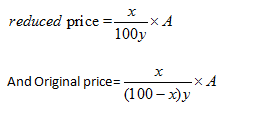Question 3: A reduction of 10% in the price enables a person to buy 2 kg more wheat for Rs 30.Find the reduced and the original price of wheat.

Solution:

Reduced Price = (x/100y)A

{(30 x 10)/(100 x 2)} Rs. = Rs. 3/2

Original Price = [x/{(100-x)y}] x  A

[(30 x 10)/{(100 – 10)x2}] = Rs. 5/3

#### Type-4: Successive profit / loss

When there are two successive profits of x% and y% then the net percentage profit =[x+y+xy/100]

When there is a profit of x% and loss of y% then net percentage profit or loss = [x – y – xy/100]

Note: If the final sign in the above expression is positive then there is net profit but if it is negative then there is net loss.

Question 4: A sells an item at a profit of 20% to B and B sells it to C at a profit of 10%. Find the resultant profit percent .

Solution: When there are two successive profit of x% and y% , net profit percentage

= {x+y+(xy/100)}

= 20+ 10 + {(20 x 10)/100} = 32%

Question 5: A sells an item at a profit of 20%to B and B sells it to C at a loss  of 10%.find the resultant profit percent.

Solution: When there are two successive profit of x% and y% net profit percentage = [x – y – xy/100]

=[20 – 10 – {(20 x 10)/100}] = 8%

#### Type-5: Goods passing through successive hands

A sells goods to B at a profit of x% and B sells it to C at a profit of y%. If C pays Rs. x for it, then the cost price for A is Rs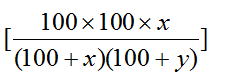Question 6: A sells a good to B at a profit of 20% and B sells it to C at a profit of 25%.If C pays Rs 222 for it, calculate the  cost price for A is

Solution:  Applying Formula(222 x 100 x 100)/(120 x 125)} = Rs. 148

Note :- For loss replace plus sign with minus .

#### Type-6: When each of the two things is sold at the same price,and a profit of p% is made on the first and a loss of L% is made on the second ,then the percentage gain or loss is: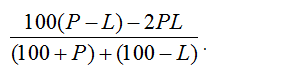Question 7. Each of the two cars is sold at the same price. A profit of 20% is made  on the first and a loss of 10% on second.calculate loss/gain %.

Apply formula :-[{100(20 – 10)-2 x 20 x 10}/{(100+20)+(100-10)}] = 20/7%

Question 8. Two items are sold for Rs 200.The first one is sold at 20% profit and second one at 20% loss. What is the % loss or gain ?

Solution : Apply FormulaAs percentage profit is equal to percentage loss so put P=L, we get,%loss = P2/100

=> %loss = (20)2/100 = 4%

#### Type-7: If cost price of x things is equal to the selling price of y articles, then profit percentage = (x-y/y)*100%

Example 9: The cost price of 18 articles is equal to the selling price of 15 articles. The gain per cent is :

Solution: Profit Percentage : (x-y/y)*100%

Gain percentage : {(18 – 15)/15} x 100

= 3/15 x 100 = 20%

Example 10: The cost price of 25 articles is equal to selling price of 20 ar­ticles. The gain percent is

Solution:

Profit percentage = (x-y/y) * 100%

%Gain = {(25-20)/20} x100 = 5/20 x 100 = 25%

### Profit and Loss Problems (Types of Questions) Practice Exercise

Question 1:
An article is sold at a loss of 10%. Had it been sold for Rs. 9 more, there would have been a gain of 25/2% on it. The cost price of the article is :
(a) Rs. 40
(b) Rs. 45
(c) Rs. 50
(d) Rs. 35

#### Answer and Explanation

option aLet the cost price of the article = Rs. x

S.P. at 10% loss = Rs 0.9x

According to question if it is sold by Rs 9 more there will be gain of 25/2 %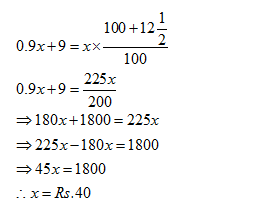Question 2:
A man sold an article at a loss of 20%. If he has sold that article for Rs. 12 more he would have gained 10%. Find the cost price of that article :
(a) Rs. 60
(b) Rs. 40
(c) Rs. 30
(d) Rs. 22

### Answer and Explanation

option bLet the cp be x

SP at 20% loss = Rs 0.8x

According to question

0.8x + 12 = 1.1x

=>0.3x = 12

=> x = Rs 40

Question 3:
If an article is sold for Rs. 178 at a loss of 11%, what should be its selling price in order to earn a profit of 11%?
(a) Rs. 222.50
(b) Rs. 267
(c) Rs. 222
(d) Rs. 220

#### Answer and Explanation

option cThe article is sold at 11% loss.

89% of CP = Rs. 178

=> CP = (178 x 100)/89 = Rs. 200

To gain 11%, S.P. = 111% of Rs. 200 = 111/100 x 200 = Rs. 222

Question 4:
A businessman sells a commodity at 10% profit. If he had bought it at 10% less and sold it for Rs. 2 less, then he would have gained 50/3%. The cost price of the commodity is
(a) Rs. 32
(b) Rs. 36
(c) Rs. 40
(d) Rs. 48

#### Answer and Explanation

option c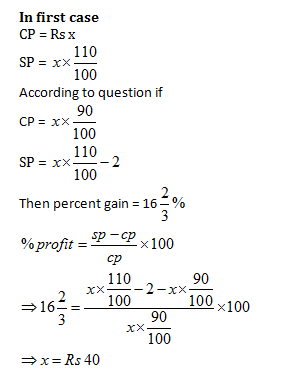Alternate solution

Let the first CP of the be Rs. 100.

First SP = Rs. 110

Second CP = Rs. 90

Gain = 50/3%

Second SP = [{90(100+50/3)}/100]= Rs. {90 x (350/300)}= Rs. 105

Difference of SPs = Rs. (110–105) = Rs. 5

Therefore If the difference in SP is Rs. 5, the CP = Rs. 100.

Therefore If the difference in SP be Rs. 2, the CP = 100/5 x 2 = Rs. 40

Question 5:
A car worth Rs. 1,50,000 was sold by X to Y at 5% profit. Y sold the car back to X at 2% loss. In the entire transaction.
(a) X gained Rs. 4,350
(b) Y lost Rs. 4,350
(c) X gained Rs. 3,150
(d) X lost Rs. 3,150

#### Answer and Explanation

error: Content is protected !!